# NCERT solutions for Chemistry Part 1 and 2 Class 11 chapter 12 - Organic Chemistry - Some Basic Principles and Techniques [Latest edition]

#### Chapters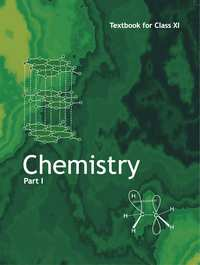## Chapter 12: Organic Chemistry - Some Basic Principles and Techniques

EXERCISES
EXERCISES [Pages 370 - 372]

### NCERT solutions for Chemistry Part 1 and 2 Class 11 Chapter 12 Organic Chemistry - Some Basic Principles and TechniquesEXERCISES [Pages 370 - 372]

EXERCISES | Q 12.1 | Page 370

What are hybridisation states of each carbon atom in the following compounds?

CH2=C=O, CH3CH=CH2, (CH3)2CO, CH2=CHCN, C6H6

EXERCISES | Q 12.2 - (a) | Page 370

Indicate the σ and π bonds in the following molecules:

C6H6

EXERCISES | Q 12.2 - (b) | Page 370

Indicate the σ and π bonds in the following molecules:

C6H12

EXERCISES | Q 12.2 - (c) | Page 370

Indicate the σ and π bonds in the following molecules:

CH2Cl2

EXERCISES | Q 12.2 - (d) | Page 370

Indicate the σ and π bonds in the following molecules:

CH= C = CH2

EXERCISES | Q 12.2 - (e) | Page 370

Indicate the σ and π bonds in the following molecules:

CH3NO2

EXERCISES | Q 12.2 - (f) | Page 370

Indicate the σ and π bonds in the following molecules:

HCONHCH3

EXERCISES | Q 12.3 - (a) | Page 370

Write bond line formulas for Isopropyl alcohol.

EXERCISES | Q 12.3 - (b) | Page 370

Write bond line formulas for 2,3-Dimethyl butanal.

EXERCISES | Q 12.3 - (c) | Page 370

Write bond line formulas for Heptan-4-one.

EXERCISES | Q 12.4 - (a) | Page 370

Give the IUPAC names of the following compound.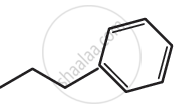EXERCISES | Q 12.4 - (b) | Page 370

Give the IUPAC names of the following compound: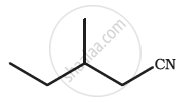EXERCISES | Q 12.3 - (c) | Page 370

Give the IUPAC names of the following compound: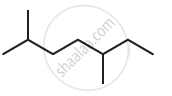EXERCISES | Q 12.4 - (d) | Page 370

Give the IUPAC names of the following compound: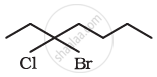EXERCISES | Q 12.4 - (e) | Page 370

Give the IUPAC names of the following compound: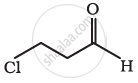EXERCISES | Q 12.4 - (f) | Page 370

Give the IUPAC names of the following compound:

Cl2CHCH2OH

EXERCISES | Q 12.5 - (a) | Page 370

Which of the following represent the correct IUPAC name for the compound concerned?

2,2-Dimethylpentane or 2-Dimethylpentane

EXERCISES | Q 12.5 - (b) | Page 370

Which of the following represent the correct IUPAC name for the compound concerned?

2,4,7-Trimethyloctane or 2,5,7-Trimethyloctane

EXERCISES | Q 12.5 - (c) | Page 370

Which of the following represent the correct IUPAC name for the compound concerned?

2-Chloro-4-methylpentane or 4-Chloro-2-methylpentane

EXERCISES | Q 12.5 - (d) | Page 370

Which of the following represent the correct IUPAC name for the compound concerned?

But-3-yn-1-ol or But-4-ol-1-yne

EXERCISES | Q 12.6 - (a) | Page 370

Draw a formula for the first five members of the homologous series beginning with the given compound.

H–COOH

EXERCISES | Q 12.6 - (b) | Page 370

Draw a formula for the first five members of the homologous series beginning with the given compound.

CH3COCH3

EXERCISES | Q 12.6 - (c) | Page 370

Draw a formula for the first five members of the homologous series beginning with the given compound.

H–CH=CH2

EXERCISES | Q 12.7 - (a) | Page 370

Give condensed and bond line structural formulas and identify the functional group(s) present, if any, for:

2,2,4-Trimethylpentane

EXERCISES | Q 12.7 - (b) | Page 370

Give condensed and bond line structural formulas and identify the functional group(s) present, if any, for

2-Hydroxy-1,2,3-propanetricarboxylic acid

EXERCISES | Q 12.7 - (c) | Page 370

Give condensed and bond line structural formulas and identify the functional group(s) present, if any, for

Hexanedial

EXERCISES | Q 12.8 - (a) | Page 370

Identify the functional group in the following compound.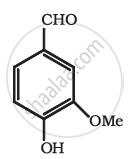EXERCISES | Q 12.8 - (b) | Page 370

Identify the functional group in the following compound.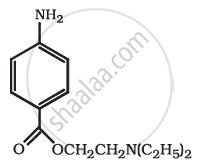EXERCISES | Q 12.8 - (c) | Page 370

Identify the functional group in the following compound.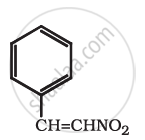EXERCISES | Q 12.9 | Page 370

Which of the two: O2NCH2CH2O or CH3CH2Ois expected to be more stable and why?

EXERCISES | Q 12.10 | Page 370

Explain why alkyl groups act as electron donors when attached to a π system

EXERCISES | Q 12.11 - (a) | Page 370

Draw the resonance structure for the following compound. Show the electron shift using curved-arrow notation.

C6H5OH

EXERCISES | Q 12.11 - (b) | Page 370

Draw the resonance structure for the following compound. Show the electron shift using curved-arrow notation.

C6H5NO2

EXERCISES | Q 12.11 - (c) | Page 370

Draw the resonance structure for the following compound. Show the electron shift using curved-arrow notation.

CH3CH = CH – CHO

EXERCISES | Q 12.11 - (d) | Page 370

Draw the resonance structure for the following compound. Show the electron shift using curved-arrow notation.

C6H5CHO

EXERCISES | Q 12.11 - (e) | Page 370

Draw the resonance structures for the following compounds. Show the electron shift using curved-arrow notation.

$\ce{C6H5 - ^+CH2}$

EXERCISES | Q 12.11 - (f) | Page 370

Draw the resonance structure for the following compound. Show the electron shift using curved-arrow notation.

$\ce{CH3CH = CH^+CH2}$

EXERCISES | Q 12.12 | Page 370

What are electrophiles and nucleophiles? Explain with examples.

EXERCISES | Q 12.13 | Page 370

Identify the reagents shown in bold in the following equations as nucleophiles or electrophiles:

a) $\ce{CH3COOH +HO- → CH3COO− + H2O}$

b) $\ce{CH3COCH3 + \bar{\text{C}}N -> (CH3)2 C(CN)(OH)}$

c) $\ce{C6H5 + CH3^+CO -> C6H5COCH3}$

EXERCISES | Q 12.14 - (a) | Page 371

Classify the following reaction in one of the reaction type studied in this unit.

$\ce{CH3CH2Br + HS– → CH3CH2SH + Br–}$

EXERCISES | Q 12.14 - (b) | Page 371

Classify the following reaction in one of the reaction type studied in this unit.

$\ce{(CH3)2 C = CH2 + HCl → (CH3)2 ClC–CH3}$

EXERCISES | Q 12.14 - (c) | Page 371

Classify the following reaction in one of the reaction type studied in this unit.

$\ce{CH3CH2Br + HO– → CH2 = CH2 + H2O + Br–}$

EXERCISES | Q 12.14 - (d) | Page 371

Classify the following reaction in one of the reaction type studied in this unit.

$\ce{(CH3)3 C – CH2 OH + HBr → (CH3)2 CBrCH2CH3 + H2O}$

EXERCISES | Q 12.15 - (a) | Page 371

What is the relationship between the members of following pairs of structures? Are they structural or geometrical isomers or resonance contributors?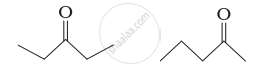EXERCISES | Q 12.15 - (b) | Page 371

What is the relationship between the members of following pairs of structures? Are they structural or geometrical isomers or resonance contributors?

$\begin{array}{cc} \ce{D}\phantom{......}\ce{H}\\ \backslash\phantom{......}/\\ \ce{C = C}\\ \phantom{...}/\phantom{......}\backslash\phantom{...}\\\ce{H}\phantom{.......}\ce{D} \end{array}$

$\begin{array}{cc} \ce{D}\phantom{......}\ce{D}\\ \backslash\phantom{......}/\\ \ce{C = C}\\ \phantom{...}/\phantom{......}\backslash\phantom{...}\\\ce{H}\phantom{.......}\ce{H}\end{array}$

EXERCISES | Q 12.15 - (c) | Page 371

What is the relationship between the members of following pairs of structures? Are they structural or geometrical isomers or resonance contributors?

$\begin{array}{cc}\ce{^+OH}\\||\\ \ce{H - C - OH}\end{array}$

$\begin{array}{cc}\ce{OH}\phantom{.}\\|\phantom{...}\\ \ce{H - C^+ - OH}\end{array}$

EXERCISES | Q 12.16 - (a) | Page 371

For the following bond cleavages, use curved arrows to show the electron flow and classify homolysis or heterolysis. Identify reactive intermediate produced as free radical, carbocation and carbanion.

$\ce{CH3O - OCH3 -> CH3\overset\bullet{\text{O}} + \overset\bullet{\text{O}}CH3}$

EXERCISES | Q 12.16 - (b) | Page 371

For the following bond cleavages, use curved-arrows to show the electron flow and classify as homolysis or heterolysis. Identify reactive intermediate produced as free radical, carbocation and carbanion.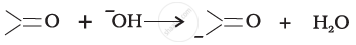EXERCISES | Q 12.16 - (c) | Page 370

For the following bond cleavages, use curved-arrows to show the electron flow and classify as homolysis or heterolysis. Identify reactive intermediate produced as free radical, carbocation and carbanion.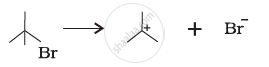EXERCISES | Q 12.16 - (d) | Page 371

For the following bond cleavages, use curved-arrows to show the electron flow and classify as homolysis or heterolysis. Identify reactive intermediate produced as free radical, carbocation and carbanion.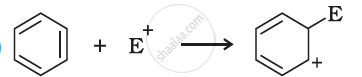EXERCISES | Q 12.17 | Page 371

Explain the terms Inductive and Electromeric effects. Which electron displacement effect explains the following correct orders of acidity of the carboxylic acids?

a) $\ce{Cl3CCOOH > Cl2CHCOOH > ClCH2COOH}$

b) $\ce{CH3CH2COOH > (CH3)2CHCOOH > (CH3)3C.COOH}$

EXERCISES | Q 12.18 - (a) | Page 371

Give a brief description of the principle of the following technique taking an example.

Crystallisation

EXERCISES | Q 12.18 - (b) | Page 371

Give a brief description of the principle of the following technique taking an example.

Distillation

EXERCISES | Q 12.18 - (c) | Page 371

Give a brief description of the principle of the following technique taking an example.

Chromatography

EXERCISES | Q 12.19 | Page 371

Describe the method, which can be used to separate two compounds with different solubilities in a solvent S.

EXERCISES | Q 12.20 | Page 371

What is the difference between distillation, distillation under reduced pressure and steam distillation ?

EXERCISES | Q 12.21 | Page 372

Discuss the chemistry of Lassaigne’s test.

EXERCISES | Q 12.22 - (i) | Page 372

Differentiate between the principle of estimation of nitrogen in an organic compound by Dumas method.

EXERCISES | Q 12.22 - (ii) | Page 372

Differentiate between the principle of estimation of nitrogen in an organic compound by Kjeldahl’s method.

EXERCISES | Q 12.23 | Page 372

Discuss the principle of estimation of halogens, sulphur and phosphorus present in an organic compound.

EXERCISES | Q 12.24 | Page 372

Explain the principle of paper chromatography.

EXERCISES | Q 12.25 | Page 372

Why is nitric acid added to sodium extract before adding silver nitrate for testing halogens?

EXERCISES | Q 12.26 | Page 372

Explain the reason for the fusion of an organic compound with metallic sodium for testing nitrogen, sulphur and halogens.

EXERCISES | Q 12.27 | Page 372

Name a suitable technique of separation of the components from a mixture of calcium sulphate and camphor.

EXERCISES | Q 12.28 | Page 372

Explain, why an organic liquid vaporises at a temperature below its boiling point in its steam distillation?

EXERCISES | Q 12.29 | Page 372

Will CCl4 give white precipitate of AgCl on heating it with silver nitrate? Give reason for your answer.

EXERCISES | Q 12.30 | Page 372

Why is a solution of potassium hydroxide used to absorb carbon dioxide evolved during the estimation of carbon present in an organic compound?

EXERCISES | Q 12.31 | Page 372

Why is it necessary to use acetic acid and not sulphuric acid for acidification of sodium extract for testing sulphur by lead acetate test?

EXERCISES | Q 12.32 | Page 372

An organic compound contains 69% carbon and 4.8% hydrogen, the remainder being oxygen. Calculate the masses of carbon dioxide and water produced when 0.20 g of this substance is subjected to complete combustion.

EXERCISES | Q 12.33 | Page 372

A sample of 0.50 g of an organic compound was treated according to Kjeldahl’s method. The ammonia evolved was absorbed in 50 mL of 0.5 M H2SO4. The residual acid required 60 mL of 0.5 M solution of NaOH for neutralisation. Find the percentage composition of nitrogen in the compound.

EXERCISES | Q 12.34 | Page 372

0.3780 g of an organic chloro compound gave 0.5740 g of silver chloride in Carius estimation. Calculate the percentage of chlorine present in the compound.

EXERCISES | Q 12.35 | Page 372

In the estimation of sulphur by Carius method, 0.468 g of an organic sulphur compound afforded 0.668 g of barium sulphate. Find out the percentage of sulphur in the given compound.

EXERCISES | Q 12.36 | Page 372

In the organic compound CH2 = CH – CH2 – CH2 – C ≡ CH, the pair of hydridised orbitals involved in the formation of: C2 – C3 bond is ______.

• sp – sp2

• sp – sp3

• sp2 – sp3

• sp3– sp3

EXERCISES | Q 12.37 | Page 372

In Lassaigne’s test for nitrogen in an organic compound, the Prussian blue colour is obtained due to the formation of ______.

• Na4[Fe(CN)6]

• Fe4[Fe(CN)6]3

• Fe2[Fe(CN)6]

• Fe3[Fe(CN)6]4

EXERCISES | Q 12.38 | Page 372

Which of the following carbocation is most stable?

• $\ce{(CH3)3C*^+CH2}$

• $\ce{(CH3)3^+C}$

• $\ce{CH3CH2^+CH2}$

• $\ce{CH3^+CH CH2C}$

EXERCISES | Q 12.39 | Page 372

The best and latest technique for isolation, purification and separation of organic compounds is ______.

• Crystallisation

• Distillation

• Sublimation

• Chromatography

EXERCISES | Q 12.40 | Page 372

The reaction: $\ce{CH_3CH_2I + KOH_{(aq)} -> CH_3CH_2OH + KI}$ is classified as ______.

• electrophilic substitution

• nucleophilic substitution

• elimination

## Chapter 12: Organic Chemistry - Some Basic Principles and Techniques

EXERCISES## NCERT solutions for Chemistry Part 1 and 2 Class 11 chapter 12 - Organic Chemistry - Some Basic Principles and Techniques

NCERT solutions for Chemistry Part 1 and 2 Class 11 chapter 12 (Organic Chemistry - Some Basic Principles and Techniques) include all questions with solution and detail explanation. This will clear students doubts about any question and improve application skills while preparing for board exams. The detailed, step-by-step solutions will help you understand the concepts better and clear your confusions, if any. Shaalaa.com has the CBSE Chemistry Part 1 and 2 Class 11 solutions in a manner that help students grasp basic concepts better and faster.

Further, we at Shaalaa.com provide such solutions so that students can prepare for written exams. NCERT textbook solutions can be a core help for self-study and acts as a perfect self-help guidance for students.

Concepts covered in Chemistry Part 1 and 2 Class 11 chapter 12 Organic Chemistry - Some Basic Principles and Techniques are Tetravalence of Carbon - Shapes of Organic Compounds, Complete, Condensed and Bond-line Structural Formulas, Three-dimensional Representation of Organic Molecules, Classification of Organic Compounds, The IUPAC System of Nomenclature, IUPAC Nomenclature of Alkanes, Nomenclature of Organic Compounds Having Functional Group(s), Nomenclature of Substituted Benzene Compounds, Fission of a Covalent Bond, Nucleophiles and Electrophiles, Electron Movement in Organic Reactions, Electron Displacement Effects in Covalent Bonds, Inductive Effect, Resonance Structure, Resonance Effect, Electromeric Effect (E Effect), Hyperconjugation, Types of Organic Reactions and Mechanisms, Methods of Purification of Organic Compounds - Introduction, Methods of Purification of Organic Compounds - Sublimation, Distillation, Methods of Purification of Organic Compounds - Differential Extraction, Methods of Purification of Organic Compounds - Chromatography, Qualitative Analysis of Organic Compounds - Detection of Carbon and Hydrogen, Quantitative Analysis of Nitrogen, Quantitative Analysis of Halogens, Quantitative Analysis of Sulphur, Quantitative Analysis of Phosphorus, Quantitative Analysis of Oxygen, Isomerism, Qualitative Analysis of Organic Compounds - Detection of Other Elements, Quantitative Analysis of Carbon and Hydrogen, Crystallization.

Using NCERT Class 11 solutions Organic Chemistry - Some Basic Principles and Techniques exercise by students are an easy way to prepare for the exams, as they involve solutions arranged chapter-wise also page wise. The questions involved in NCERT Solutions are important questions that can be asked in the final exam. Maximum students of CBSE Class 11 prefer NCERT Textbook Solutions to score more in exam.

Get the free view of chapter 12 Organic Chemistry - Some Basic Principles and Techniques Class 11 extra questions for Chemistry Part 1 and 2 Class 11 and can use Shaalaa.com to keep it handy for your exam preparation